# R軟體再談行導向

> (x <- matrix(1:24,8,3))

[,1]  [,2]  [,3]

[1,]    1    9    17

[2,]    2   10   18

[3,]    3   11   19

[4,]    4   12   20

[5,]    5   13   21

[6,]    6   14   22

[7,]    7   15   23

[8,]    8   16   24

> (y <- apply(x, 2, min))

  1  9 17

> (z <- apply(x, 2, max))
 8 16 24

y 與z 看起來像是列向量，實際上它們是行向量！因此x 須先轉置(transpose)，才能將各行剪去其最小值。

>  t(x) - y

[ ,1]  [,2]  [,3]  [,4]  [,5]  [,6]  [,7]  [,8]

[1,]    0    1     2     3    4     5     6    7

[2,]    0    1     2     3    4     5     6    7

[3,]    0    1     2     3    4     5     6    7

> x - y
[,1]   [,2]   [,3]

[1,]     0    -8      8

[2,]    -7     9      1

[3,]  -14     2     18

[4,]     3    -5     11

[5,]    -4    12      4

[6,]  -11     5      21

[7,]    6     -2     14

[8,]   -1    15       7

> (t(x) - y)/(z - y)

[,1]           [,2]             [,3]             [,4]             [,5]            [,6]             [,7]  [,8]

[1,]     0  0.1428571  0.2857143  0.4285714  0.5714286  0.7142857  0.8571429    1

[2,]     0  0.1428571  0.2857143  0.4285714  0.5714286  0.7142857  0.8571429    1

[3,]     0  0.1428571  0.2857143  0.4285714  0.5714286  0.7142857  0.8571429    1

> t*1

[,1]            [,2]             [,3]

[1,] 0.0000000  0.0000000  0.0000000

[2,] 0.1428571  0.1428571  0.1428571

[3,] 0.2857143  0.2857143  0.2857143

[4,] 0.4285714  0.4285714  0.4285714

[5,] 0.5714286  0.5714286  0.5714286

[6,] 0.7142857  0.7142857  0.7142857

[7,] 0.8571429  0.8571429  0.8571429

[8,] 1.0000000  1.0000000  1.0000000

 撰文者： 鄒慶士 博士 現任： 北商資訊與決策科學所教授 中華 R 軟體學會理事長 信箱：

*1:t(x) - y)/(z - y

# 巨人的肩膀與 plyr R軟體套件

plyr 的讀音同英文的老虎鉗(pliers)去掉字尾 s，顧名思義它是運用分割-應用-組合(split-apply-combine)的策略於資料分析的工作上，也就是說將一大的問題，切割為可管控的子集，個別進行問題解決後，再重新組合起來。舉例來說，先建一個包含三個數值變數與兩個因子的72 筆資料，

> library(plyr)

> dd <- data.frame(matrix(round(rnorm(216), 2), 72, 3), c(rep("A", 24), rep("B", 24), rep("C", 24)), c(rep("J", 36), rep("K", 36)))

> colnames(dd) <- c("v1", "v2", "v3", "dim1", "dim2")

v1        v2       v3   dim1   dim2
1     0.32    -0.55    2.52         A        J
2    -1.13     1.27   -0.19         A        J
3     0.40     1.51   -0.30         A        J
4     0.40     0.65    1.37         A        J
5    -0.93     0.67   -0.09         A       J
6    -0.05     0.17    0.71         A       J

plyr 套件中的 ddply 函數可以不用撰寫迴圈，迅速地計算出兩因子 dim1 與 dim2 各種可能組合下，數值變數 v1 的平均數。

> ddply(dd, c("dim1", "dim2"), function(df) mean(df\$v1))

dim1   dim2                 V1
1        A        J    -0.05333333
2        B        J    -0.15416667
3        B        K     0.14666667
4        C        K     0.17041667

> ddply(dd, c("dim1", "dim2"), summarise, V1 = mean(v1))

dim1   dim2                 V1
1        A        J    -0.05333333
2        B        J    -0.15416667
3        B        K     0.14666667
4        C        K     0.17041667

ddply 的頭兩個英文字母分別代表函數的輸入為資料框(data frame)，輸出結果亦為資料框。當然，plyr 套件還有其它的函數，ldply 表輸入為串列(list)，輸出為資料框；alply 表輸入為陣列(array)，輸出為串列；d_ply 則不傳回任何物件，通常此函數輸出到圖形裝置或檔案，更多的資訊請參考 plyr 手冊或下面的參考資料。

 撰文者： 鄒慶士 博士 現任： 北商資訊與決策科學所教授 中華 R 軟體學會理事長 信箱：

# R軟體應用於機率密度曲線與累積分配曲線的繪製

> pnorm(1.5, 0, 1)

 0.9331928

> qnorm(0.9331928, 0, 1)

 1.5

> curve(dnorm(x, 0, 1), xlim = c(-3, 3), main = "Area to left of 1.5 = pnorm(1.5, 0, 1)")

> cord.x <- c(-3, seq(-3, 1.5, 0.01), 1.5)

> cord.y <- c(0, dnorm(seq(-3, 1.5, 0.01)), 0)

> polygon(cord.x, cord.y, col = "grey")

> text(-0.5, 0.1, "area=pnorm(1.5,0,1)")

>text(1.5, 0.02, "1.5=qnorm(0.9331928, 0 ,1)")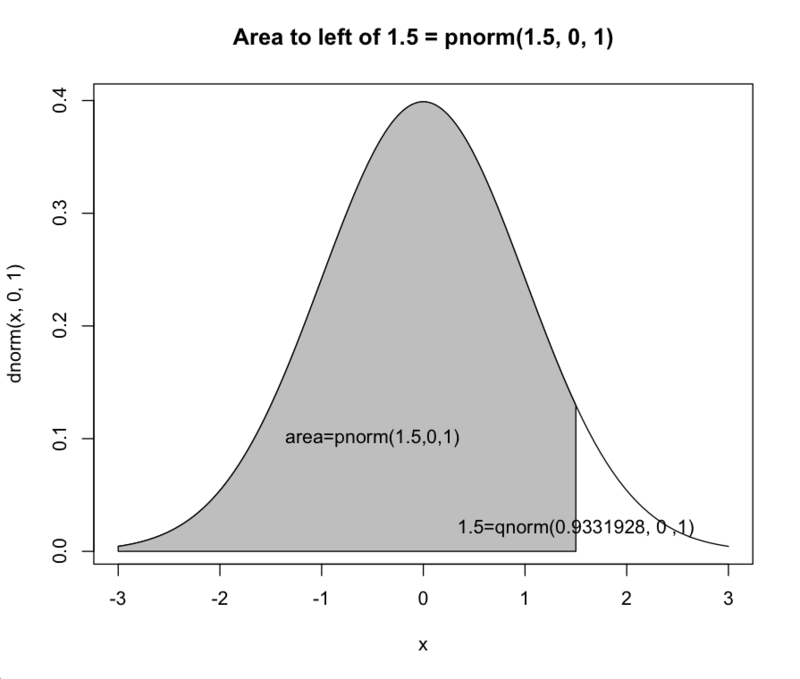> curve(pnorm(x, 0, 1), xlim = c(-3,3), main = "F(z)=P(Z<=z)")

> abline(h = 0.9331928, lty = 2)

> abline(v = 1.5, lty = 3)

> text(-2, 0.85, "pnorm(1.5) is 0.9331928")

> text(1.5, 0.02, "qnorm( 0.9331928) is 1.5")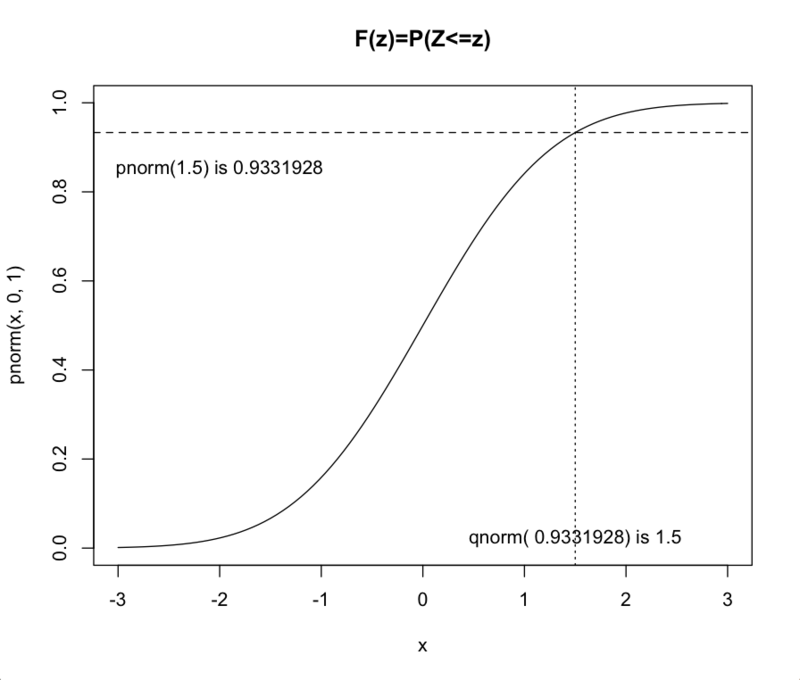參考資料：

Verzani, J. (2001), simpleR &ndash; Using R for Introductory Statistics, The CSI MathDepartment, City University of New York.

 撰文者： 鄒慶士 博士 現任： 北商資訊與決策科學所教授 中華 R 軟體學會理事長 信箱：

# R軟體的寬資料與長資料

> mydata <- data.frame(var1 = c(12, 15, 19, 22, 15), var2 = c(18, 12, 42, 29, 44), var3 = c(8, 17, 22, 19, 31))

>mydata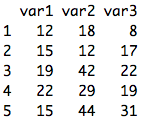> sdata <- stack(mydata)

> sdata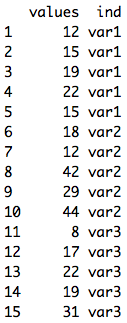stack 函數還可使用select 參數選擇要轉換成長資料的變數，此處我們選var1 和var2。

> sdata <- stack(mydata, select = c(var1, var2))

>sdata\$in == "var3"

 FALSE FALSE FALSE FALSE FALSE FALSE FALSE FALSE FALSE FALSE

> mydata <- unstack(sdata, values ~ ind)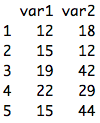參考資料：Spector, P. (2008), Data Manipulation with R, Springer.

 撰文者： 鄒慶士 博士 現任： 北商資訊與決策科學所教授 中華 R 軟體學會理事長 信箱：

# R軟體for迴圈的迷思

> (a <- 1:5)

 1 2 3 4 5

> (b <- 5:1)

 5 4 3 2 1

> d <- NULL

> for ( i in 1:length(a)) {

+ d[i] <- a[i] * b[i]

+ }

>d

 5 8 9 8 5

> s <- 0

> for (i in 1:length(d)) {

+ s <- s+d[i]

+ }

> s

 35

> (d <- a * b)

 5 8 9 8 5

> (s <- sum(a * b)

 35

 撰文者： 鄒慶士 博士 現任： 北商資訊與決策科學所教授 中華 R 軟體學會理事長 信箱：

# R軟體apply系列基本函數的運用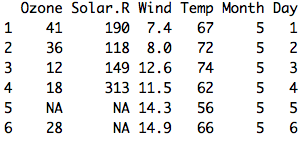> apply(airquality, 2, mean) # apply(資料及名稱, 2表逐行,套用函數名稱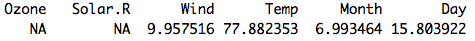> apply(airquality, 2, mean, na.rm=T)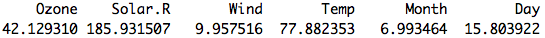apply 系列的基本函數還有lapply 與sapply，lapply 將airquality 資料視為六個元素的串列(list)，逐一元素加總遺缺值個數，故無須加入逐列或逐行的參數值。

> lapply(airquality, function(x) sum(is.na(x)))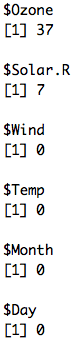sapply 可將上面結果以較簡單的矩陣形式展現，此即為函數名字首的含義。

> sapply(airquality, function(x) sum(is.na(x)))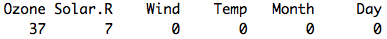sapply 函數也可以判斷哪些變數是因子，或加入自定函數計算因子變數的水準數，讀者當可想出apply系列基本函數的其它應用。最後，善用apply 方可將R 程式技巧提升到更高的層次。

>sapply(InsectSprays, is.factor)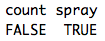> sapply(insectSprays, sunction(x) if (!is.factor(x)) return(0) else length(levels(x)))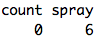參考資料：

Maindonald, J.H. (2004), Using R for Data Analysis and Graphics: Introduction, Code,and Commentary, http://www.stats.uwo.ca/DAAG/.

 撰文者： 鄒慶士 博士 現任： 北商資訊與決策科學所教授 中華 R 軟體學會理事長 信箱：

# R軟體如何儲存隨機種子？

R是一個很好的模擬工具，有許多內建函數可資運用。使用者有時希望獲得相同的模擬結果，則可將隨機種子(random seed)儲存起來重複利用。例如：

> set.seed(12)
> seed <- .Random.seed
> x <- runif(10)
> .Random.seed <- seed
> y <- runif(10) x-y

 0 0 0 0 0 0 0 0 0 0

> # seesion 1

> set.seed(10)

> seed <- .Random.seed

> x<-runif(10)

> write.table(x, "x")

>write.table(seed, "seed")

> q( )

> # session 2

> .Random.seed <- t(seed)

> y <- runif(10)

> x - y

x

1       3.330669e-16

2       3.330669e-16

3       -2.220446e-16

4       -4.440892e-16

5       2.775558e-17

6       -1.110223e-16

7       2.220446e-16

8       -1.665335e-16

9       -3.330669e-16

10     1.110223e-16

 撰文者： 鄒慶士 博士 現任： 北商資訊與決策科學所教授 中華 R 軟體學會理事長 信箱：